# Question: Identify the conjugate acid of CO32- and theconjugate base of HSO4- in the followingreaction: HSO4-(aq) + CO32-(aq)=HCO3-(aq) +SO42-(aq)

A. H2CO3 and H2SO4
B. HCO3 and SO42-
C. HCO3 and H2SO4
D. H2CO3 and HSO4
E. none of the above

```Transcribed text:
A. H2CO3 and H2SO4
B. HCO3- and SO42-
C. HCO3- and H2SO4
D. H2CO3 and HSO4-
E. none of the above```
Contents

`Answer:`

General guidance:

Concepts and reason

The concept used to solve this problem is determined Conjugated acid-Base.Fundamentals

Bronsted-Lowry acid-base system:

Acid:

According to Bronsted-Lowry; acid is a substance that donates the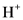(hydrogen ion) or proton. In general Bronsted-Lowry acid is a proton donor.

Base:

According to Bronsted-Lowry; base is a substance that accepts the(hydrogen ion) or proton. In general Bronsted-Lowry base is a proton acceptor.

After donate one proton from an acid conjugate base will form; thus,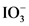is the conjugate base of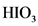.

After accepts one proton by a base conjugate acid will form; thus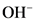is the conjugate acid of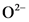.

Step-by-step:

### Step 1 of 2

The conjugate acid of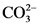will form after accepting one proton as follows:

Explanation | Hint for next step

The conjugate acid ofis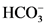because the acid of conjugated acid-base pair has one more hydrogen atom from base of conjugated acid-base pair.

### Step 2 of 2

The conjugate base of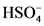will from after donating one proton as follows:

Therefore, in the given reaction; conjugate acid and conjugate base are as follow:

Thus, the conjugate acid is  and conjugate base is .
Explanation

The pair of Acid-Base which is differs by a proton is called conjugated Acid-Base pair. The acid of conjugated acid-base pair has one more hydrogen atom from base of conjugated acid-base pair, while base of conjugated acid-base pair has one less hydrogen atom from acid of conjugated acid-base pair.

Thus, the conjugate acid isand conjugate base is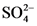.
```Faq: